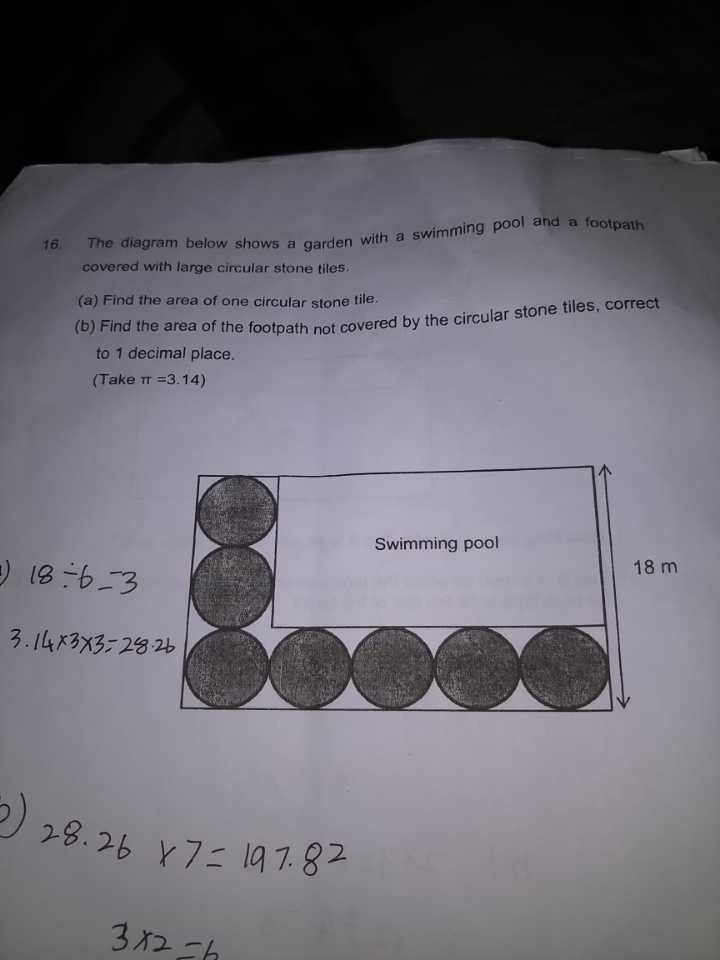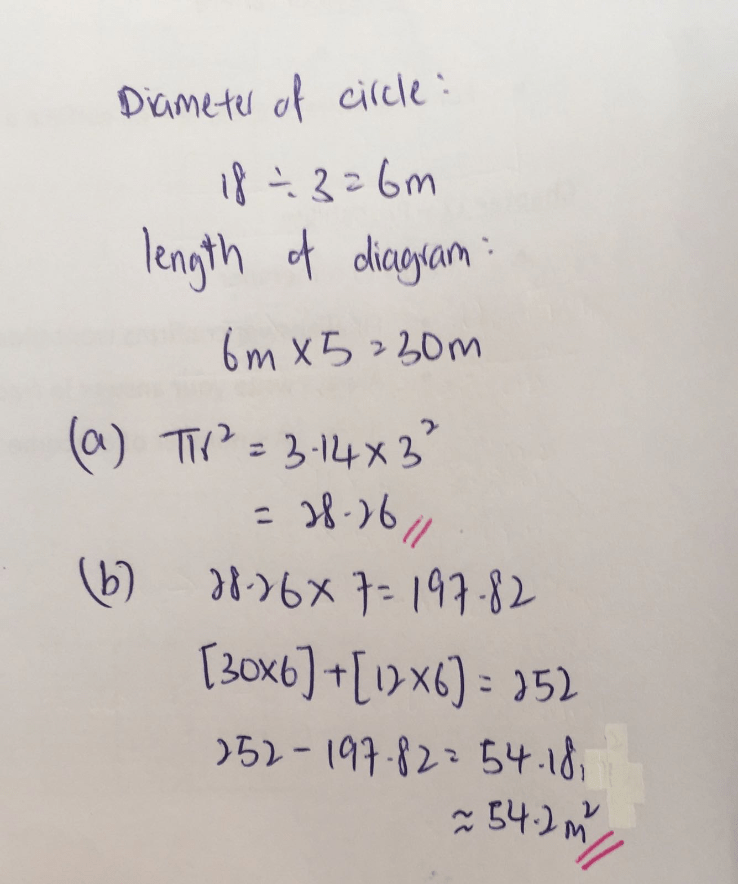# Question(a)
18/3 = 6 (diameter of circular stone tile)
3.14 x (6/2) x (6/2) = 28.26

(b)

6 x 6 – 28.26) x 7 = 54.18 = 54.2 (correct to 1 dp)

or

6 x 6 x 7 = 252
28.26 x 7 = 197.82
252 – 197.82 = 54.18 = 54.2 (correct to 1 dp)

Ans : (a) 28.26 sq m; (b) 54.2 sq m (correct to 1 dp).

0 Replies 0 Likes